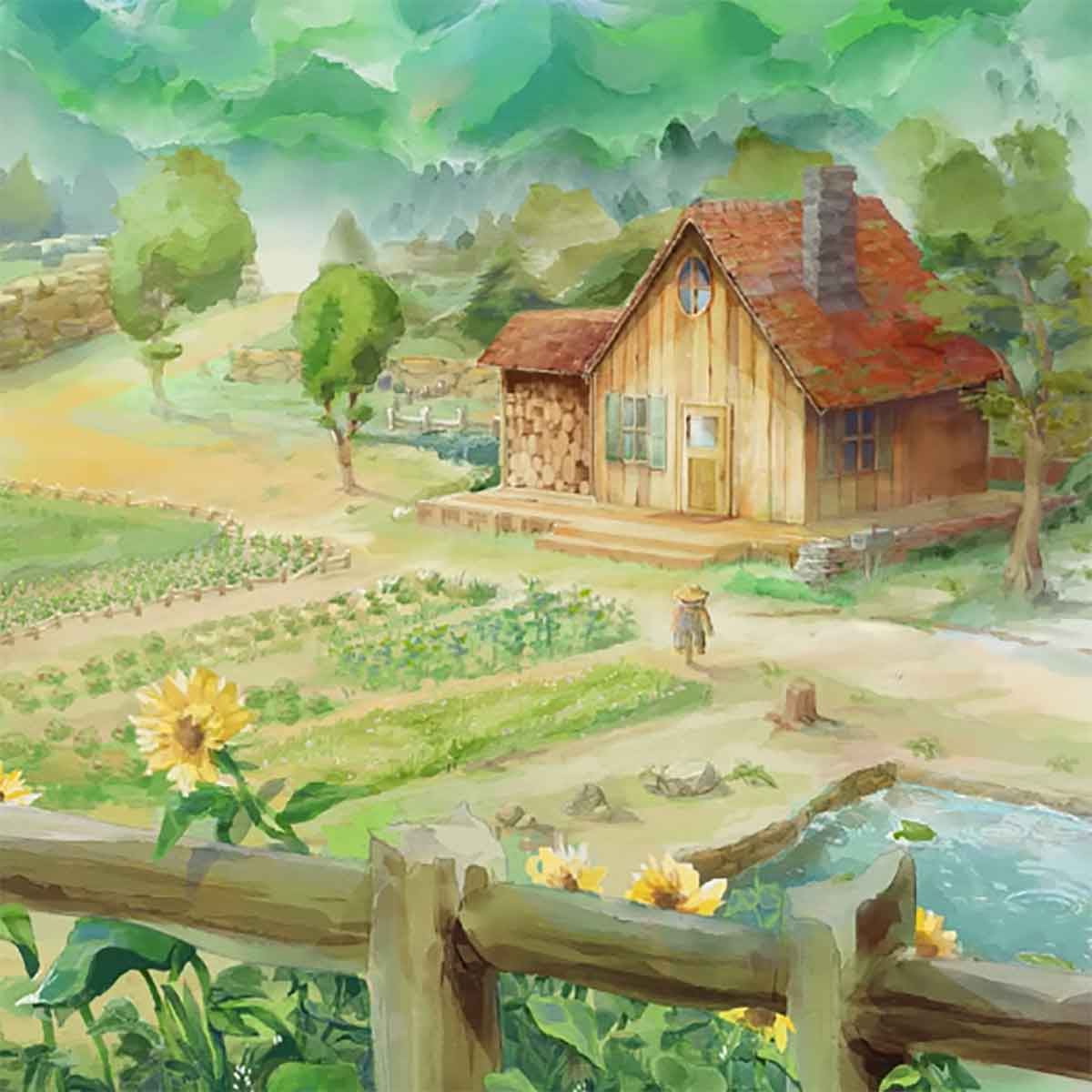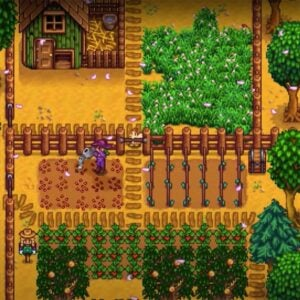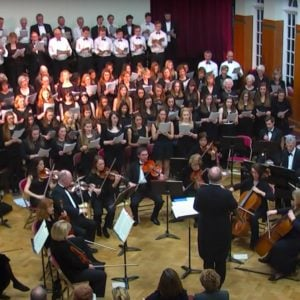# Overture (Stardew Valley)

11 November 2020
5
01:54
• ##### TEMPO
96
0
(0)

7 ( Q Qr r7 0 W Wr r7 ( Q Qr r[YD7]| |7 ( Q Qr r7 0 W Wr r7 ( Q Qr r7| |[D7] ( Q Q[rG]| [f7] 0 [WD] W[rf]| [G7] ( Q Q[rD]| [a7] ( Q Qr| [D7] ( Q Q[rG]| [f7] 0 [WH] W[rG]| [S\$] ^ * *Q| \$ ^ * Q E Q [D7] ( Q Q[rG]| [f7] 0 [WD] W[rf]| [G7] ( Q Q[rD]| [a7] ( Q Qr S [D7] ( [SQ] Q[ra]| [O7] 0 W W[rI]| [a7] ( Q Qr| 7 ( Q r Y I [I7]aD( Q Q[rG]| [f7]Gf0 [WD] W[rf]| [G7] ( Q Q[rD]| [a7] ( Q Qr| [I7]aD( Q Q[rG]| [f7] 0 [WH] W[rG]| [I\$]PS^ * *Q| \$ ^ * Q E Q [I7]aD( Q Q[rG]| [f7]Gf0 [WD] W[rf]| [G7] ( Q Q[ra]| [H7] 0 [WG] W[rf]| [D7] ( [SQ] Q[ra]| [O7] 0 W W[rI]| [a7] ( Q ( r Q Y r I Y a P [rk] Y I a I [YJ] [rH] u O a O [uf] [rG] Y I a I [YD] [SQ] E T I [aT] [SE] [rD] Y I a [OI] Y [rf] u [OD] a [fO] u [rG] Y I a IG[YJ]k[QC] E T I T E [rk] Y I a I [YJ] [rH] u O a [fO] u [rG] Y I a I [YH] [QJ] E T I T [GE] [k(] W r Y r [WL] [J(] Q E T [GE] Q [rk] Y I a I Y r Y I a I Y [rG7]DSaGDSaGDSa[D7]SaIDSaIDSaI[aW%]POYaPOYaPOY[O30]IurOIurOIur[rQ7]rY

Rate This Music Sheet:

Average rating 0 / 5. Vote count: 0

No votes so far! Be the first to rate this music sheet.

Thank you for rating this song!

If you have any specific feedback about how to improve this music sheet, please submit this in the box below.

Overture (Stardew Valley) is a song by Eric Barone. Use your computer keyboard to play Overture (Stardew Valley) music sheet on Virtual Piano. This is an Intermediate song and requires a lot of practice to play well. The recommended time to play this music sheet is 01:54, as verified by Virtual Piano legend, Arda. The song Overture (Stardew Valley) is classified in the genre of Songs From Games on Virtual Piano. You can also find other similar songs using Instrumental.

## Other Songs By Eric Barone

•[a8]|s|[wd]|f|[a8] s d f [wa]|p|[a8]|s|[wd]|f|[a8]sas d f [wa]|p|[a6]|s|[hd0]|f|[da6] s d f [fa0]|p|[wtoe6] | [a8]|s|[wd]|f|[a8] s d [kf] [wha]|p d [fa8]|s|[wd]|f|[a8]sas d [kf] [wha]|p d [sp6]|a|[d0]|f|[a6] s d h [z0] j h k [l4] k h d [s8] a o u [tq4] | [t8]|a|i|u|p|a|i|[us] y [ta8]|a|i|u|p|a|i|[up] y [tsqe6]|a|i|u|p|a|i|[uo] y [tqea]|a|i|u|p|a|d | [ta8]|a|[oi]|u|[to]|a s [od] f a k [sh8]|a|i|u|r|a s [od] f h k [lh6] k h d [us] a o u [tp]|a|u|y|[tq4] | t | [tq4]|p|a|s|[a8]|s|[wd]|f|[a8] s d [kf] [wha]|p d [fa8]|s|[wd]|f|[a8]sas d [kf] [wha]|p d [sp6]|a|[d0]|f|[a6] s d h [z0] j h k [l4] k h d [s8] a o u [tq4] | [uto8] | z|l | [uoa0] | h|G | [sqpi] | c|x | j|[uto8] | [uto8] | 5|[shf8]|f|d|f|h | x|m|[khf0]|h|G|h|k | b|n|[qljg]|j|h|g|h | s | [d8] f r t y|u|o|p|opo|[y5]|[uto81]|u|y|u|[uto] | [xl]|l|[uoa30]|o|I|o|[uoa] | [jf]|k|[sqpi4]|p|o|i|[uo] | t | [t81]|r t y|u|o|p|opo|[y5]|[t81]

Level: 6
Length: 02:45
Intermediate

## Related

•s d [sf] h g [sg] j [hd] [sh] l [kd] [lf] h f s d f [pg] h [ja] [sh] g f [pd] f s [oa] s d o a d [ga] f d [sf] s d f h g [sg] j [hd] [sh] l [kd] [lf] h f s d f p h g f d s o s [ia] [us] f h l h f s f h [tl8] u| r| [ie]| [ro]| e| [wuo]| [ie]| r| [ut]| [yw] o p [yoa] d [ws] [wda] g [wf] g d a o a d [ga] f d [sf] s d f h [ga] [sg] j [hd] [sh] l [kd] [lf] h f s d f p h g f d s o s a s f d f h g [sg] j [hd] [sh] l [kd] [lf] h f s d f [pg] h [ja] [sh] g f [pd] f s [oa] s d o a d [ga] f d [sf] s d f h g [sg] j [hd] [sh] l [kd] [lf] h f s d f p h g f d s o s [ia] [uts]| r| [ie]| [ro]| e| [wuo]| [ie]| r| [ut]| [yw] o p [yoa] d [wsp] [wda] g [wf] g d a o a d [ga] f d [sf] s d f h g [sg] j [hd] [sh] l [kd] [lf] h f s d f p h g f d s o s [ia] [us] f d [sf] h g [sg] j [hd] [sh] l [kd] [lf] h f s d f [pg] h [ja] [sh] g f [pd] f s [oa] s d o a d [ga] f d [sf] s d f h g [sg] j [hd] [sh] l [kd] [lf] h f s d f p h g f d s o s [ia] s f h l h f [so] f [pG] [ha] o p [oa] d S S f d [pd] g f g d p i o p p h g h f S p a S [pd] g f [gd] j h [hd] J j [jg] z [fL] [zg] j g [dP] f g [dJ] j h [ga] f d p d S d g j z
Level: 6
Length: 02:06
Intermediate

#### Johann Sebastian Bach

•h f s d s| |f|||o|| [toe]o[up] p p u t [y0] o a a [so] [ya] [ys] u p p u t [r0] y [so] [od] [ys] [sr] [tf] u [pf] p u t y [ro] [yo] o [yo] [ro] [tpe] u p p u t [y0] r [yp] [so] [yp] [rp] [tse] u p p u t [y0] r [yo] o [yo] [ro] [tpe] u p p u t [y0] r y [of] [yf] [rf] [tfe]d[us] [sp] p u t [r0] y [po] [so] [yp] [rp]o [tpe] u p p u t [ur0] [yo] [po] [po] [ys] [sr] [se]ap [pe] e f [ed] [f0]ds 0 0|[o0] [p6]|6 6 s [p6] [sp0]|[u0] [o0] p [oa0] [se]ap [pe] e f [ed] [f0]ds 0 0oo [o0] [p6]|6 6 s [p6] [s0] h||j 3 [j6] 6h[f6] [d6]fds 6 3|p [sp] p [p3]o [p6]o[p6]s6 6|6 3sds[d3]s[d3]s [f6] 6 6 6|[f6] 3 oo o [o3] [o3] [p6] 6 [u6] [p6]|[u6] [o3] p 3 [f3] f [f3]|| [f6]d[s6] [s6] 6|6 3 ppps p [p3]o [p6]o[p6]s6 6|6 3|h j [j3]3 [j6]h[f6] [h6] [f6]ds [f6] [d3]|o o [o3] [o3] [sf6] [sp6] [a6] [p6] a [s6] [u3] [o3] [p3] [p3] [a3] [s3] [s6]ap p 6 f [d6] [f3]d[s3] 3 3 3 [o3] [p6]||6 s [p6] [s3] 3 [u3] [o3] [p3] [a5] [s6]ap p 6 f [d6] [f3]d[p3] 3 3o[o3] [o3] [p6]||6 s [p6] [s3] 3 3 [o3] [o3] [o3] [u6]||p|6 [o3]||a|3 [s6]||p|6 [u3]||[o3] [p3] 3 [spf6]
Level: 5
Length: 02:09
Intermediate

#### Alicia Keys

•a o a d s a p a | p I p s a p o p | o u o a p o I o I o p I y u I y | a o a d s a p a p I p s a p o p o u o a p o I o | j g j g k h k h l j l j z k z k x l x l x l x l | l j l k l k h h x z l k l k h l j l k l k h j j g j h j h s | a p a p a p s a | u o u o p a s a p o p o u p s p s d f g f d s p d s d s | e r t y a a p a a p a a p a p u t o p i p a O a a p | o p h f f g h j J j h g g f g d f g d f g h H h g f o p s s o p s s x l f g h l | h f s l J l J g J j f g h j h f h f s l J l J g J j f g h | p p a a s s S S c x z l z l j J J l J j J l b v v c c x c x z | f S S d a S S S p o a f S S d a S S S p o a h k z C C v C V b b V b | 6
Level: 3
Easy